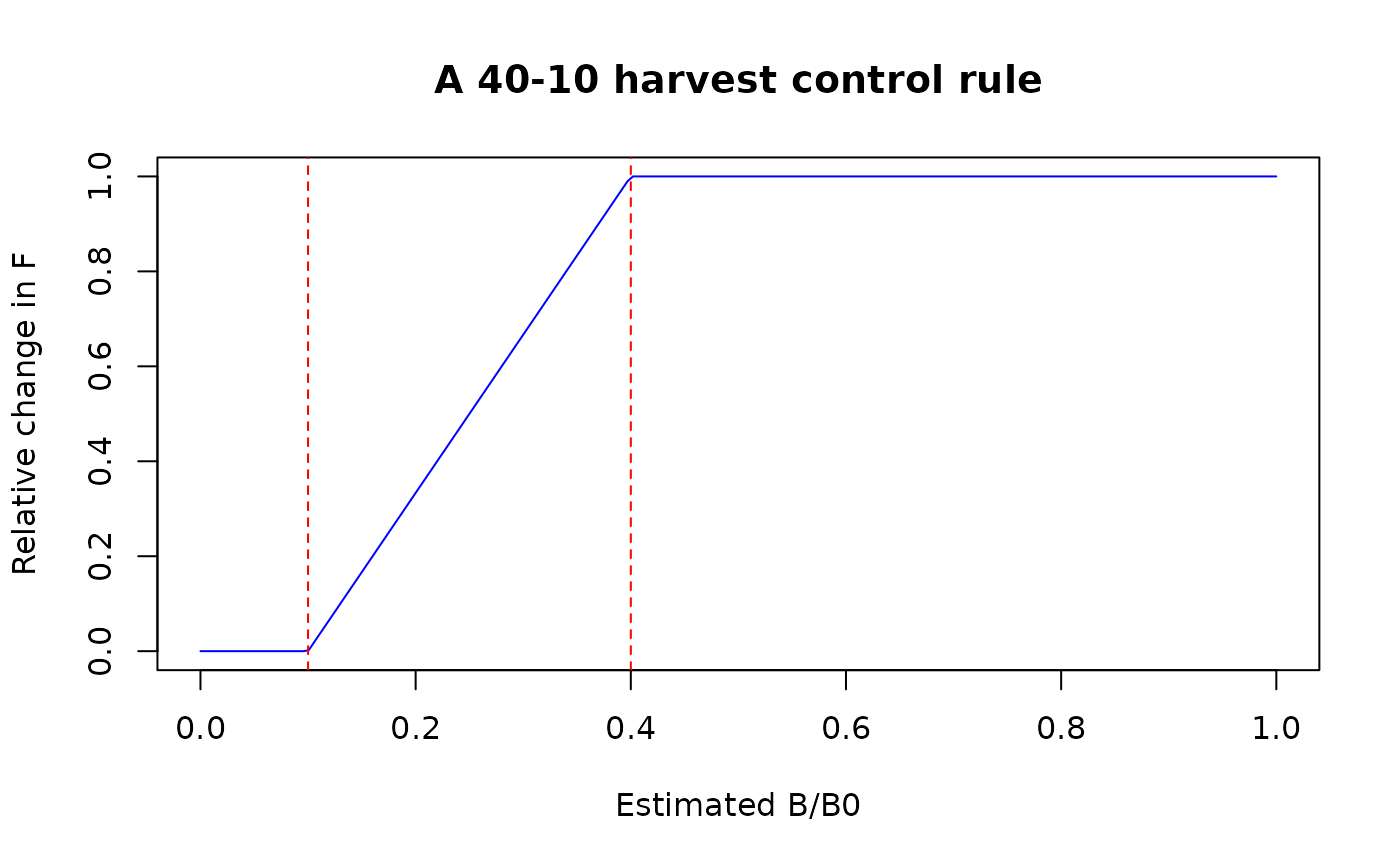A general function used by HCR_ramp that adjusts the output (e.g., F) by a linear ramp based on the value of the OCP relative to target and limit values.

HCRlin(OCP_val, LOCP, TOCP, relF_min = 0, relF_max = 1)

## Arguments

OCP_val

The value of the operational control point (OCP).

LOCP

Numeric, the limit value for the OCP in the HCR.

TOCP

Numeric, the target value for the OCP in the HCR.

relF_min

The relative maximum value (e.g. a multiple of FMSY) if OCP < LOCP.

relF_max

The relative maximum value (e.g. a multiple of FMSY) if OCP > TOCP.

T. Carruthers

## Examples

#40-10 linear ramp
Brel <- seq(0, 1, length.out = 200)
plot(Brel, HCRlin(Brel, 0.1, 0.4), xlab = "Estimated B/B0", ylab = "Relative change in F",
main = "A 40-10 harvest control rule", type = 'l', col = 'blue')
abline(v = c(0.1,0.4), col = 'red', lty = 2)